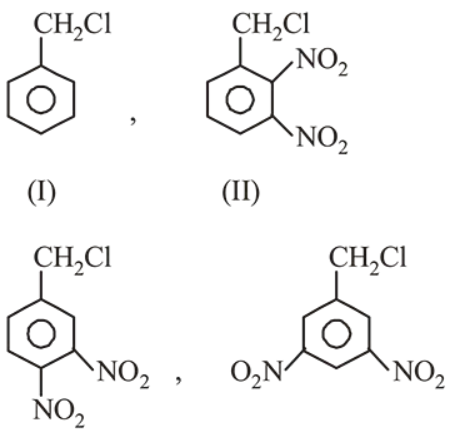# The decreasing order of reactivity of the followingQuestion:

The decreasing order of reactivity of the following compounds towards nucleophilic substitution $\left(\mathrm{S}_{\mathrm{N}} 2\right)$ is :1. (II) $>$ (III) $>$ (I) $>$ (IV)

2. (II) $>$ (III) $>$ (IV) $>$ (I)

3. (III) $>$ (II) $>$ (IV) $>$ (I)

4. (IV) $>$ (II) $>$ (III) $>$ (I)

Correct Option: , 2

Solution:

$\mathrm{S}_{\mathrm{N}} 2$ reactions depend upon $-\mathrm{I}$ and $-\mathrm{M}$ effect on substrate. On increasing - I and -M effect, rate of $\mathrm{S}_{\mathrm{N}} 2$ reaction will increase.